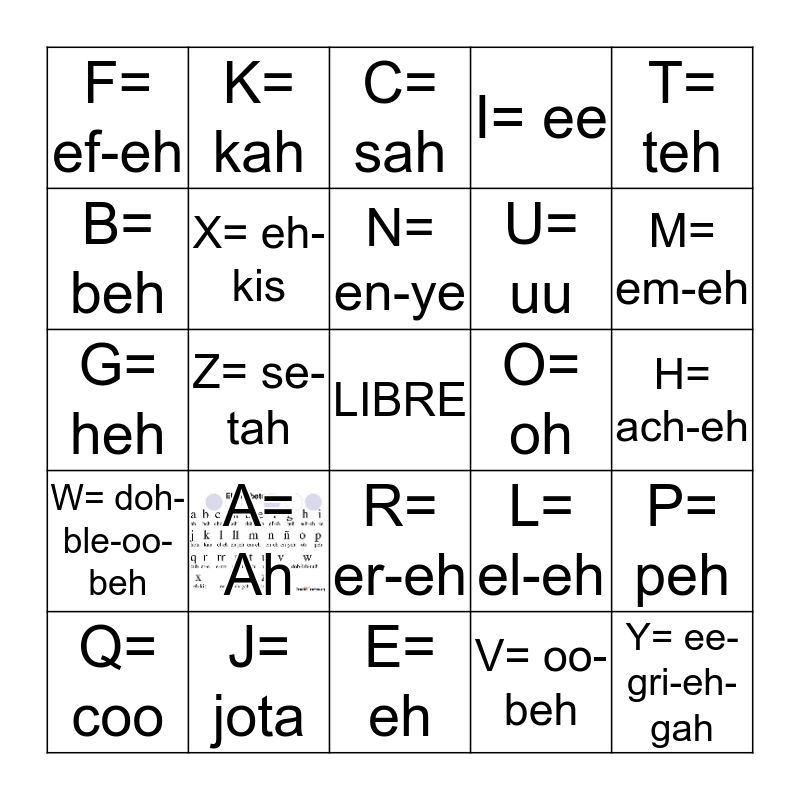This bingo card has 1 images, a free space and 24 words: A= Ah, J= jota, N= en-ye, Q= coo, T= teh, F= ef-eh, B= beh, K= kah, O= oh, R= er-eh, U= uu, G= heh, L= el-eh, P= peh, X= eh- kis, V= oo-beh, H= ach-eh, C= sah, M= em-eh, Z= se-tah, Y= ee-gri-eh-gah, W= doh-ble-oo-beh, I= ee and E= eh.

## Play Online